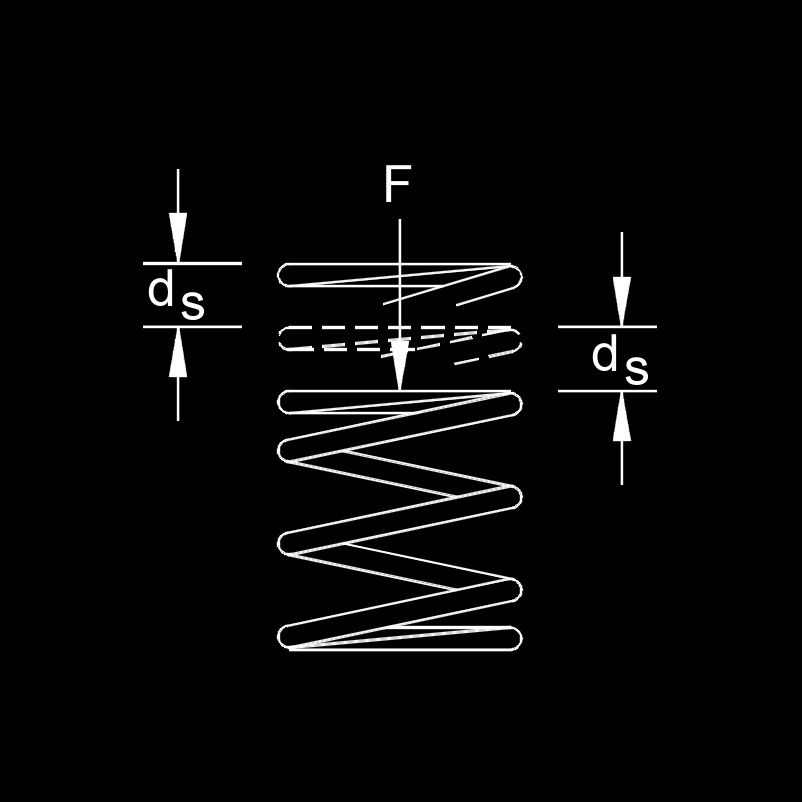# Spring Displacement

Written by Jerry Ratzlaff on . Posted in FastenerSpring displacement, abbreviated as $$d_s$$, also called spring deformed, spring deflection or travel distance, is the compression (a repulsive force) or stretching (an attractive force) of a spring.

## Spring Displacement formulas

 $$\large{ d_s = \sqrt { \frac {2 \; E_s}{ k_s } } }$$ $$\large{ d_s = \frac {F_s }{ k_s } }$$

### Where:

$$\large{ d_s }$$ = spring displacement

$$\large{ k_s }$$ = spring constant

$$\large{ E_s }$$ = spring energy

$$\large{ F_s }$$ = spring force